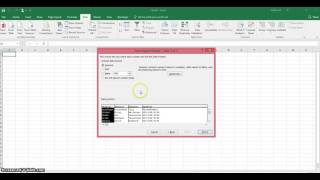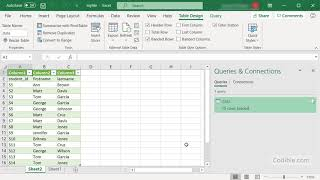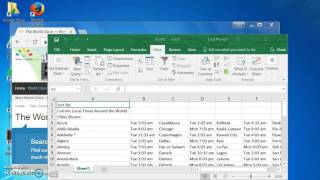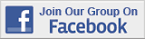Excel Tips & Tutorials:
 Suggest a Link Alphabetize Page Printer-Friendly List
Watch and Learn
Watch and Learn
Watch and Learn
Watch and Learn
 Import Data from WebLeila Gharani demonstrates wih oil price and weather examples (10:01).How To Import A Text FileKeith Aul shows how to import data from plain text file (9:14).Import Tab Delimited Text FileShows how to work with common data format (3:35)Importing Data from a Website to SpreadsheetLucy Provost demonstrates Excel 2016 web queries (6:38)Import Data from WebLeila Gharani demonstrates wih oil price and weather examples (10:01).How To Import A Text FileKeith Aul shows how to import data from plain text file (9:14).Import Tab Delimited Text FileShows how to work with common data format (3:35)Import Data from WebLeila Gharani demonstrates wih oil price and weather examples (10:01).How To Import A Text FileKeith Aul shows how to import data from plain text file (9:14).Import Data from WebLeila Gharani demonstrates wih oil price and weather examples (10:01).RegressIt: Excel Add-On for Data Analysis Performs multivariate descriptive data analysis and regression analysis with high-quality table and chart output in...SolverStat SolverStat is an Excel add-in (xla) that performs advanced statistical tests on least squares regression data.RegressIt: Excel Add-On for Data Analysis Performs multivariate descriptive data analysis and regression analysis with high-quality table and chart output in...SolverStat SolverStat is an Excel add-in (xla) that performs advanced statistical tests on least squares regression data.RegressIt: Excel Add-On for Data Analysis Performs multivariate descriptive data analysis and regression analysis with high-quality table and chart output in...SolverStat SolverStat is an Excel add-in (xla) that performs advanced statistical tests on least squares regression data.RegressIt: Excel Add-On for Data Analysis Performs multivariate descriptive data analysis and regression analysis with high-quality table and chart output in...Over 18,000 Members!